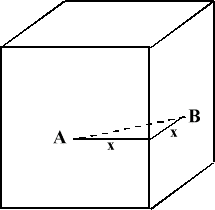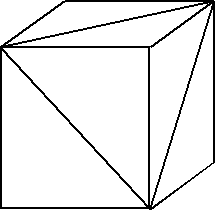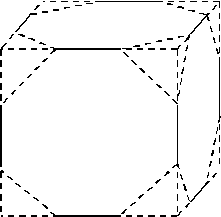#### You may also like### Some(?) of the Parts

A circle touches the lines OA, OB and AB where OA and OB are perpendicular. Show that the diameter of the circle is equal to the perimeter of the triangleA 1 metre cube has one face on the ground and one face against a wall. A 4 metre ladder leans against the wall and just touches the cube. How high is the top of the ladder above the ground?### At a Glance

The area of a regular pentagon looks about twice as a big as the pentangle star drawn within it. Is it?

# Three Cubes

##### Age 14 to 16Challenge Level

Thank you Andrew Clark for this solution.

1. The distances between the centres of two adjacent faces of another cube is $8$cms. What is the side length of this cube?Let the side length of the cube be $2x$. From the centre points A and B of both faces to the line at which their planes meet is $x$, and AB ($= 8$) is the hypotenuse of a right angled triangle whose other two sides have length $x$ . Therefore $2x^2 = 8^2$ , so that $x= \sqrt{32}$ , and the length of the side is $8\sqrt{2}$ .

2. It is known that the area of the largest equilateral triangular section of a cube is $140$cm$^2$ . What is the side length of the cube?Cube with equilateral triangle section.

Let the sides of the equilateral triangle have length $a$; then the area of the triangle is $(a/2)(a\sqrt{3}/2) = a^2\sqrt{3}/4$. As the area of the triangle is $140 {\rm cm}^2$, $a^2 = 560/\sqrt{3}$. The length of the diagonal of a face of the cube is $a$; let $b$ be the length of a side of the cube. Then, by Pythagoras' Theorem, $2b^2 = 560/\sqrt{3}$ so that $b=12.7$ to three significant figures.

3. Another cube has an edge length of $12$cms. At each vertex a tetrahedron with three mutually perpendicular edges of length $4$cms is sliced away. What is the surface area and volume of the remaining solid?From each face of the cube we remove four triangles whose total area is $32 {\rm cm}^2$ . Thus the area left is $144-32 = 112 {\rm cm}^2$ . There are six such faces giving an area of $672 {\rm cm}^2$ . \par In addition, there are eight equilateral triangular faces of side length $4\sqrt{2}$ . The total area of these is $8 \times 8\sqrt{3} = 64\sqrt{3} {\rm cm}^2$ . Thus the total surface area is $672 + 64\sqrt{3} = 783 {\rm cm}^2$ to the nearest square centimetre.

The volume of a tetrahedron is $(1/3) \times \hbox{(area of base)} \times \hbox{height}$ . Consider one of the tetrahedra, and take one of the right-angled triangles as its base; then its volume is $(1/3) \times 8 \times 4 = 32/3$ . Thus the volume of the remaining solid is $12^3 - (8\times 32)/3 = 1643 {\rm cm}^3$ to the nearest cubic centimetre.